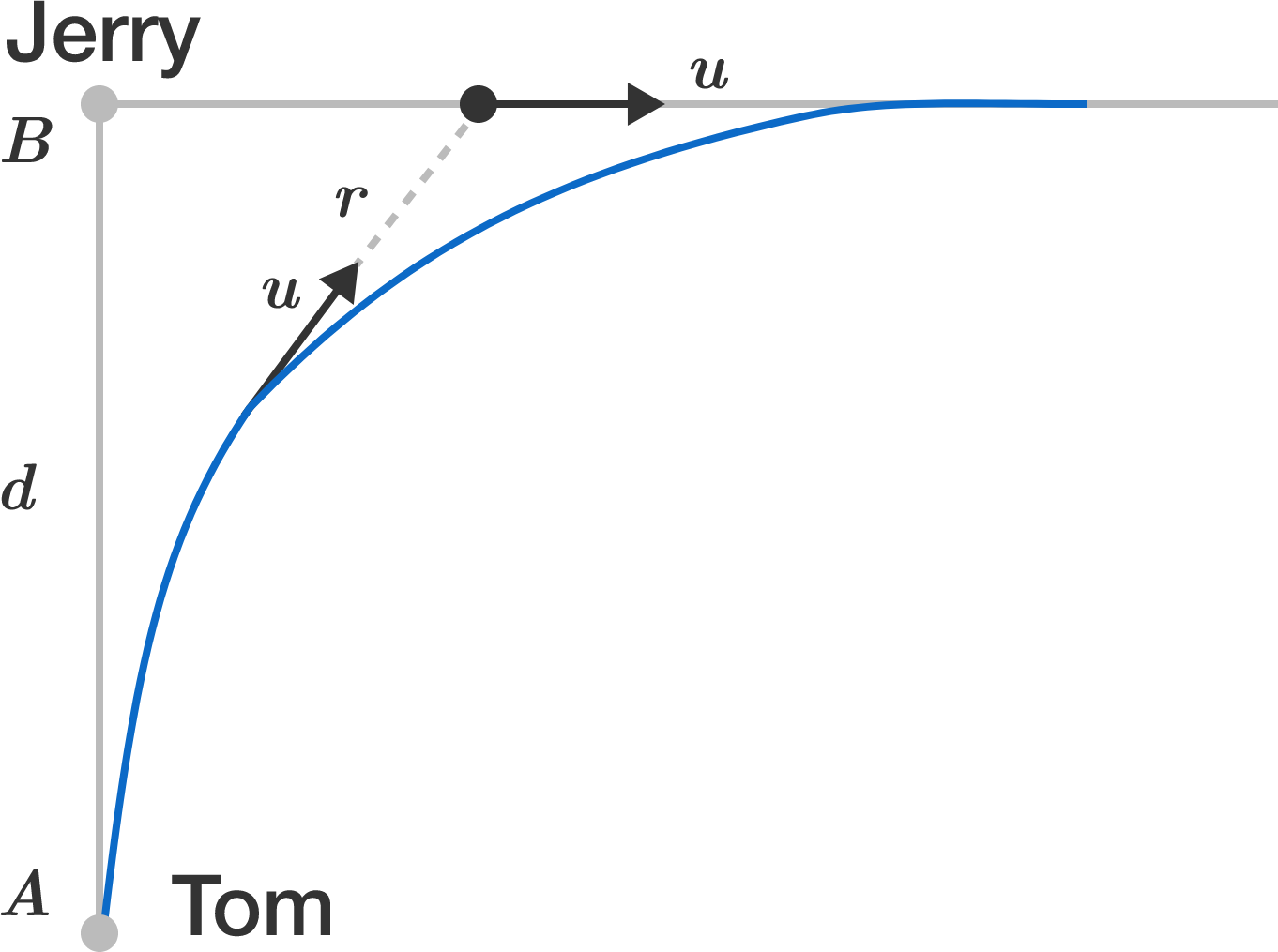# Separation at $t=\infty$

Tom and Jerry both have equal top running speeds and are initially at points $A$ and $B,$ respectively, separated by a distance of $d$.

They both spot each other and immediately start running at their top speeds. Jerry runs on a straight line perpendicular to the line $AB$ and Tom runs in such a way that its velocity always points towards the current location of Jerry.

Let $r(t)$ denote the distance between Tom and Jerry at time $t$.

Find $\displaystyle \lim_{t \to \infty} r(t)$.×